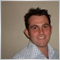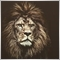# changing extern input setting318

Hello anyone that can help.

I would like to ad some programming to my EA to make it really simple for the user. I would like to have default settings for low, medium and high risk.

e.g. if the user sets the "extern bool" to true for "UseRiskPresets"

and the "extern RiskType = LowRisk

then all the external settings for stop loss, take profit and lot size would update according to my programming. I have written the function for the different risk types but unsure how to link them to the external settings.

thanks much appreciated9849

Hello anyone that can help.

I would like to ad some programming to my EA to make it really simple for the user. I would like to have default settings for low, medium and high risk.

e.g. if the user sets the "extern bool" to true for "UseRiskPresets"

and the "extern RiskType = LowRisk

then all the external settings for stop loss, take profit and lot size would update according to my programming. I have written the function for the different risk types but unsure how to link them to the external settings.

thanks much appreciated318

Lakshan Perera:

//+------------------------------------------------------------------+

//|                               http://www.boafx.com |

//+------------------------------------------------------------------+

#property version   "1.00"

#property strict

#include <stderror.mqh>

#include <stdlib.mqh>

enum RiskType{LowRisk,MediumRisk,HighRisk};

extern bool UseRiskPresets=true;

extern RiskType Risk_Type=LowRisk;//Which Type Of risk to use?

extern int MacdFastEma = 12;

extern int MacdSlowEma = 26;

extern int Threshold = 10;

extern double StopLoss=0;

extern double TakeProfit=50;

input double   LotSize=0.1;//The Lotsize you would like to use.

input double   Maxlotsize=1.0;

extern int MagicSeed=1000;//Number from which to compute MagicNumber

int magic;

double pips;

//+------------------------------------------------------------------+

//| Expert initialization function                                   |

//+------------------------------------------------------------------+

int OnInit()

{

//---Generate a magic number based on our MagicSeed

magic = MagicNumberGenerator();

// Determine what a pip is.

pips =Point; //.00001 or .0001. .001 .01.

if(Digits==3||Digits==5)

pips*=10;

//---

#include<InitChecks.mqh>

//---

return(INIT_SUCCEEDED);

}

//+------------------------------------------------------------------+

//| Expert deinitialization function                                 |

//+------------------------------------------------------------------+

void OnDeinit(const int reason)

{

//---

}

//+------------------------------------------------------------------+

//| Expert tick function                                             |

//+------------------------------------------------------------------+

void OnTick()

{

for (int i=100;i>0;i--){

Comment(i);}

if(UseRiskPresets)RiskPresets();

static datetime candletime = 0;

if(candletime != Time)

{

CheckForSignal();

candletime = Time;

}

}

//+------------------------------------------------------------------+

//|       TRIGGER FUNCTION                                                           |

//+------------------------------------------------------------------+

void CheckForSignal()

{

//---gather signal data

double CurrentMacd = iMACD(NULL,0,MacdFastEma,MacdSlowEma,9,PRICE_CLOSE,MODE_MAIN,1);

double PreviousMacd = iMACD(NULL,0,MacdFastEma,MacdSlowEma,9,PRICE_CLOSE,MODE_MAIN,2);

double PreviousPreviousMacd = iMACD(NULL,0,MacdFastEma,MacdSlowEma,9,PRICE_CLOSE,MODE_MAIN,3);

//---test for sell signal

if(Low<Close && CurrentMacd < PreviousMacd && PreviousMacd > PreviousPreviousMacd && CurrentMacd > +Threshold*pips)

if(TotalOpenSellOrders())

{

}

else if (High>Close && CurrentMacd > PreviousMacd && PreviousMacd < PreviousPreviousMacd && CurrentMacd < -Threshold*pips)

{

}

}

//+------------------------------------------------------------------+

//+------------------------------------------------------------------+

int err=0;

double price=Bid,

sl=0,

tp=0,

maxlotsize = Maxlotsize,

lotsize = (TotalOpenSellOrders()+1) * LotSize;

if(lotsize>maxlotsize)

lotsize=LotSize;//this sets the lotsize to the preset

{

}

//----

int ticket =  OrderSend(Symbol(),type,lotsize,price,30,0,0,"EaTemplate",magic,0,Magenta);

if(ticket>0)

{

if(OrderSelect(ticket,SELECT_BY_TICKET))

{

if(OrderType() == OP_SELL)

{

sl = OrderOpenPrice()+(StopLoss*pips);

if(StopLoss==0)sl=0;

tp = BreakevenOfSells()-(TakeProfit*pips);

}

{

sl = OrderOpenPrice()-(StopLoss*pips);

if(StopLoss==0)sl=0;

}

//---Modify This Order

if(!OrderModify(ticket,price,sl,tp,0,Magenta))

{

err = GetLastError();

Print("Encountered an error during modification!"+(string)err+" "+ErrorDescription(err)  );

}

//---Modify The Other Orders that are Open.

if(TotalOpenOrders() > 1)

{

for(int i=0;i<OrdersTotal()-1;i++)

{

if(OrderMagicNumber()==magic)

if(OrderType()==type)

if(!OrderModify(OrderTicket(),OrderOpenPrice(),OrderStopLoss(),tp,0,clrMagenta))

{

err = GetLastError();

Print("Encountered an error during modification!"+(string)err+" "+ErrorDescription(err)  );

}

}

}

}

else

{//in case it fails to select the order for some reason

Print(__FUNCTION__,"Failed to Select Order ",ticket);

err = GetLastError();

Print("Encountered an error while seleting order "+(string)ticket+" error number "+(string)err+" "+ErrorDescription(err)  );

}

}

else

{//in case it fails to place the order and send us back a ticket number.

err = GetLastError();

Print("Encountered an error during order placement!"+(string)err+" "+ErrorDescription(err)  );

}

}

//+------------------------------------------------------------------+

//|Generate a Magic Number                                           |

//+------------------------------------------------------------------+

int MagicNumberGenerator()

{

string mySymbol=StringSubstr(_Symbol,0,6);

int pairNumber=0;

int GeneratedNumber=0;

else if(mySymbol == "AUDCHF")    pairNumber=2;

else if(mySymbol == "AUDJPY")    pairNumber=3;

else if(mySymbol == "AUDNZD")    pairNumber=4;

else if(mySymbol == "AUDUSD")    pairNumber=5;

else if(mySymbol == "CHFJPY")    pairNumber=8;

else if(mySymbol == "EURAUD")    pairNumber=9;

else if(mySymbol == "EURCHF")    pairNumber=11;

else if(mySymbol == "EURGBP")    pairNumber=12;

else if(mySymbol == "EURJPY")    pairNumber=13;

else if(mySymbol == "EURNZD")    pairNumber=14;

else if(mySymbol == "EURUSD")    pairNumber=15;

else if(mySymbol == "GBPAUD")    pairNumber=16;

else if(mySymbol == "GBPCHF")    pairNumber=18;

else if(mySymbol == "GBPJPY")    pairNumber=19;

else if(mySymbol == "GBPNZD")    pairNumber=20;

else if(mySymbol == "GBPUSD")    pairNumber=21;

else if(mySymbol == "NZDJPY")    pairNumber=23;

else if(mySymbol == "NZDCHF")    pairNumber=24;

else if(mySymbol == "NZDUSD")    pairNumber=25;

else if(mySymbol == "USDCHF")    pairNumber=27;

else if(mySymbol == "USDJPY")    pairNumber=28;

else if(mySymbol == "XAGUSD")    pairNumber=29;

else if(mySymbol == "XAUUSD")    pairNumber=30;

else if(mySymbol == "SPX500")    pairNumber=31;

else if(mySymbol == "AUS200")    pairNumber=32;

//do the 5 character cfd's

else mySymbol=StringSubstr(mySymbol,0,5);

if(mySymbol      == "GER30")     pairNumber=33;

else if(mySymbol == "FRA40")     pairNumber=34;

//do the 4 characther cfd's

else mySymbol=StringSubstr(mySymbol,0,4);

if(mySymbol == "US30")      pairNumber=35;

GeneratedNumber=MagicSeed+(pairNumber*1000)+_Period;

return(GeneratedNumber);

}

//+------------------------------------------------------------------+

//Total of ALL orders place by this expert.

//+------------------------------------------------------------------+

int TotalOpenOrders()

{

int total=0;

for(int i=OrdersTotal()-1; i >= 0; i--)

{

if(OrderMagicNumber()== magic)

total++;

else Print(__FUNCTION__,"Failed to select order ",GetLastError());

}

return (total);

}

//+------------------------------------------------------------------+

//Total of all SELL orders place by this expert.

//+------------------------------------------------------------------+

int TotalOpenSellOrders()

{

int total=0;

for(int i=OrdersTotal()-1; i >= 0; i--)

{

{

if(OrderMagicNumber()== magic)

if(OrderType() == OP_SELL)

total++;

}

else Print(__FUNCTION__,"Failed to select order ",i," ",GetLastError());

}

return (total);

}

//+------------------------------------------------------------------+

//Total of all BUY orders place by this expert.

//+------------------------------------------------------------------+

{

int total=0;

for(int i=OrdersTotal()-1; i >= 0; i--)

{

{

if(OrderMagicNumber()== magic)

total++;

}

else Print(__FUNCTION__,"Failed to select order",GetLastError());

}

return (total);

}

//+------------------------------------------------------------------+

//| find the price of the highest sell that is open                  |

//+------------------------------------------------------------------+

double HighestSellPosition()

{

double HighestOpenPrice=0.0;

for (int cnt=OrdersTotal()-1; cnt >= 0; cnt--)

{

if(OrderMagicNumber()== magic)

if(OrderType()==OP_SELL)

if(OrderOpenPrice()>HighestOpenPrice)

HighestOpenPrice=OrderOpenPrice();

}

return(HighestOpenPrice);

}

//+------------------------------------------------------------------+

//| find the price of the lowest buy that's open.                    |

//+------------------------------------------------------------------+

{

double LowestOpenPrice=0.0;

for (int cnt=OrdersTotal()-1; cnt >= 0; cnt--)

{

if(OrderMagicNumber()== magic)

if(LowestOpenPrice==0.0)

LowestOpenPrice=OrderOpenPrice();

else if (OrderOpenPrice()<LowestOpenPrice)

LowestOpenPrice=OrderOpenPrice();

}

return(LowestOpenPrice);

}

//+------------------------------------------------------------------+

//| Calculates Breakeven of Open Sell Orders.                                                                  |

//+------------------------------------------------------------------+

double BreakevenOfSells()

{

double TotalLots = 0,

price = 0,

lots =0,

pricetimeslots =0,

Total =0,

TotalPriceTimesLots=0;

for(int cnt=0;cnt<OrdersTotal();cnt++)

{

if (OrderMagicNumber()== magic)

if(OrderType()==OP_SELL)

{

price = OrderOpenPrice();

lots = OrderLots();

pricetimeslots = price * lots;

TotalLots += lots;

TotalPriceTimesLots += pricetimeslots;

}

}

Total = TotalPriceTimesLots/TotalLots;

return(Total);

}

//+------------------------------------------------------------------+

//| Calculates Breakeven of Open Buy Orders.                                                                  |

//+------------------------------------------------------------------+

{

double TotalLots = 0,

price = 0,

lots =0,

pricetimeslots =0,

Total =0,

TotalPriceTimesLots=0;

for(int cnt=0;cnt<OrdersTotal();cnt++)

{

if (OrderMagicNumber()== magic)

{

price = OrderOpenPrice();

lots = OrderLots();

pricetimeslots = price * lots;

TotalLots += lots;

TotalPriceTimesLots += pricetimeslots;

}

}

Total = TotalPriceTimesLots/TotalLots;

return(Total);

}

//+------------------------------------------------------------------+

//|SET RISK MANAGMENT                                                  |

//+------------------------------------------------------------------+

void RiskPresets()

{

switch(Risk_Type)

{

case LowRisk: LowRisk();return;

case MediumRisk: MediumRisk();return;

case HighRisk: HighRisk();return;

default: return;

}

}

//+------------------------------------------------------------------+

//|  LowRisk presets                                                                |

//+------------------------------------------------------------------+

void LowRisk()

{

double Threshold = 10;

double TakeProfit=6;

double   LotSize=0.01;//The Lotsize you would like to use.

double   Maxlotsize=0.1;

return;

}

//+------------------------------------------------------------------+

//|  MediumRisk presets                                                                |

//+------------------------------------------------------------------+

void MediumRisk()

{

double Threshold = 20;

double TakeProfit=15;

double   LotSize=0.02;//The Lotsize you would like to use.

double   Maxlotsize=0.3;

return;

}

//+------------------------------------------------------------------+

//| HighRisk presets                                                                |

//+------------------------------------------------------------------+

void HighRisk()

{

double Threshold = 30;

double TakeProfit=25;

double   LotSize=0.04;//The Lotsize you would like to use.

double   Maxlotsize=1.1;

return;

}9849

Next time use this Code button to insert the codes.. it is difficult to read..
remove  "double" in your functions.. because you are not re declaring the input variables..

```void HighRisk()
{
Threshold=30;
TakeProfit=25;
LotSize=0.04;//The Lotsize you would like to use.
Maxlotsize=1.1;
return;
}```

Do this for all of your 3 functions..

and also change the following to externs..

```input double   LotSize=0.1;//The Lotsize you would like to use.
input double   Maxlotsize=1.0;

//Change the above input to extern

extern double   LotSize=0.1;//The Lotsize you would like to use.
extern double   Maxlotsize=1.0;```

And just so you know, now you can directly set the SL and TP for the orders in the OrderSend() function..318

Lakshan Perera:

Next time use this Code button to insert the codes.. it is difficult to read..
remove  "double" in your functions.. because you are not re declaring the input variables..

Do this for all of your 3 functions..

and also change the following to externs..

And just so you know, now you can directly set the SL and TP for the orders in the OrderSend() function..

thank you very much I'll give it a go